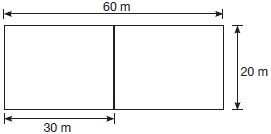# User ForumSubject :IMO    Class : Class 3

In the given figure, a large rectangle is 60 metres long and 20 metres wide. 2 small rectangles are 30 metres long and 20 metres wide each. Which of the following statements is CORRECT?A The total length of the large rectangle is twice the total length of the small rectangle.
B The total length of the large rectangle is equal to the total length of the small rectangle.
C The total length of the large rectangle is 30 meters more than the total length of the small rectangle.
D The total length of the large rectangle is equal to 160 meters and the total length of the small rectangle is equal to 100 meters.

option A and C are both correct. If D is the correct option then instead of length you should make it more specific to say length of the boundary. A rectangle by definition has 2 sides length and breadth

## Ans 1:

Class : Class 2
both A and C are correct

Class : Class 7

## Ans 3:

Class : Class 5
Please use the correct wordings, Perimeter. Itâs just confusing to deal with word length.

## Ans 4:

Class : Class 7
A and C are correct.Please write unambiguous lines. Write boundary or perimeter to be precise.as children view rectangle in length and breadth only.

Class : Class 5
A

## Ans 6:

Class : Class 3
Both A and C are correct

## Ans 7:

Class : Class 2
sorry I was wrong the ans is d only because total length is here equal to perimeter for this condition

## Ans 8:

Class : Class 5
A and C both are correct. it should be perimeter or boundary.

## Ans 9:

Class : Class 3
lenght should be replaced by parameter. ques.wording is wrong

## Ans 10:

Class : Class 6
The correct answer is D because the total length of the large rectangle is 160 meters and the total length of the small rectangle is 100 meters

## Ans 11:

Class : Class 3
The word should be PERIMETER as the word TOTAL LENGTH [mentioned] can mean the sum of the 2 sides of the rectangle and can not be understood very well.So, according to me the answer can be both A as well as C.